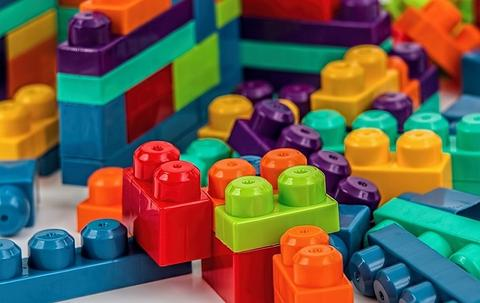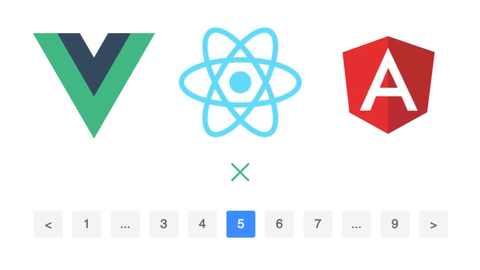DevUI是一支兼具设计视角和工程视角的团队，服务于华为云DevCloud平台和华为内部数个中后台系统，服务于设计师和前端工程师。

Ng组件库：ng-devui（欢迎Star）

## 计算方法

`M = E − N + 2P`

E 为图中边的个数

N 为图中节点的个数

P 为连接组件的个数

## 度量工具

### ESLint

`eslint`也可以配置关于圈复杂度的规则，如：

``````rules: {
complexity: [
'error',
{
max: 15
}
]
}``````

## 常用结构圈复杂度

### 顺序结构

``````function func() {
let a = 1, b = 1, c;
c = a * b;
}
``````

``M = 1 - 2 + 2 * 1 = 1``

### if-else-else、switch-case

``````function func() {
let a = 1, b = 1, c;
if (a = 1) {
c = a + b;
} else {
c = a - b;
}
}``````

``M = 4 - 4 + 2 * 1 = 2``

### 循环结构

``````function func() {
let a = 1, b = 1, c = 2;
for (let i = 1; i < 10; i++) {
b += a;
}
c = a + b;
}``````

``M = 4 - 4 + 2 * 1 = 2``

## 常用降低模块（函数）圈复杂度方法

### 1. 函数提炼与拆分，单一职责（推荐）

``````function add(a, b) {
let tempA;
if (a === 10) {
tempA = 11;
} else if (a === 12) {
tempA = 12;
}
let tempB;
if (b === 10) {
tempB = 13;
} else if (b === 12) {
tempB = 12;
}
return tempB + tempA;
}``````

``````function add(a, b) {
return calcA(a) + calcB(b);
}

function calcA(a) {
if (a === 10) {
return 11;
} else if (a === 12) {
return 12;
}
}

function calcB(b) {
if (b === 10) {
return 13;
} else if (b === 12) {
return 12;
}
}``````

### 2. 优化算法（减少不必要条件、循环分支）

``````let a = 'a', c;
if (a === 'a') {
c = a + 1;
} else if (a === 'b') {
c = a + 2;
} else if (a === 'c') {
c = a + 3;
} else if (a === 'd') {
c = a + 4;
}
return c;``````

``````let a = 'a', c;
let conditionMap = {
a: 1,
b: 2,
c: 3,
d: 4
}
c = a + conditionMap[a];
return c;
``````

### 3. 表达式逻辑优化

``a && b || a && c``

``a && (b || c)``

### 5. 减少提前return（此方法需辩证看待）

``````let a = 1, b = 1;
if (a = 1) {
return a + b;
} else {
return a - b;
}``````

``````let a = 1, b = 1, c;
if (a = 1) {
c = a + b;
} else {
c = a - b;
}
return c;``````

## 加入我们

oa gina：手把手教你搭建自己的Angular组件库zhuanlan.zhihu.comoa gina：手把手教你使用Vue/React/Angular三大框架开发Pagination分页组件zhuanlan.zhihu.comoa gina：手把手教你搭建一个灰度发布环境zhuanlan.zhihu.com